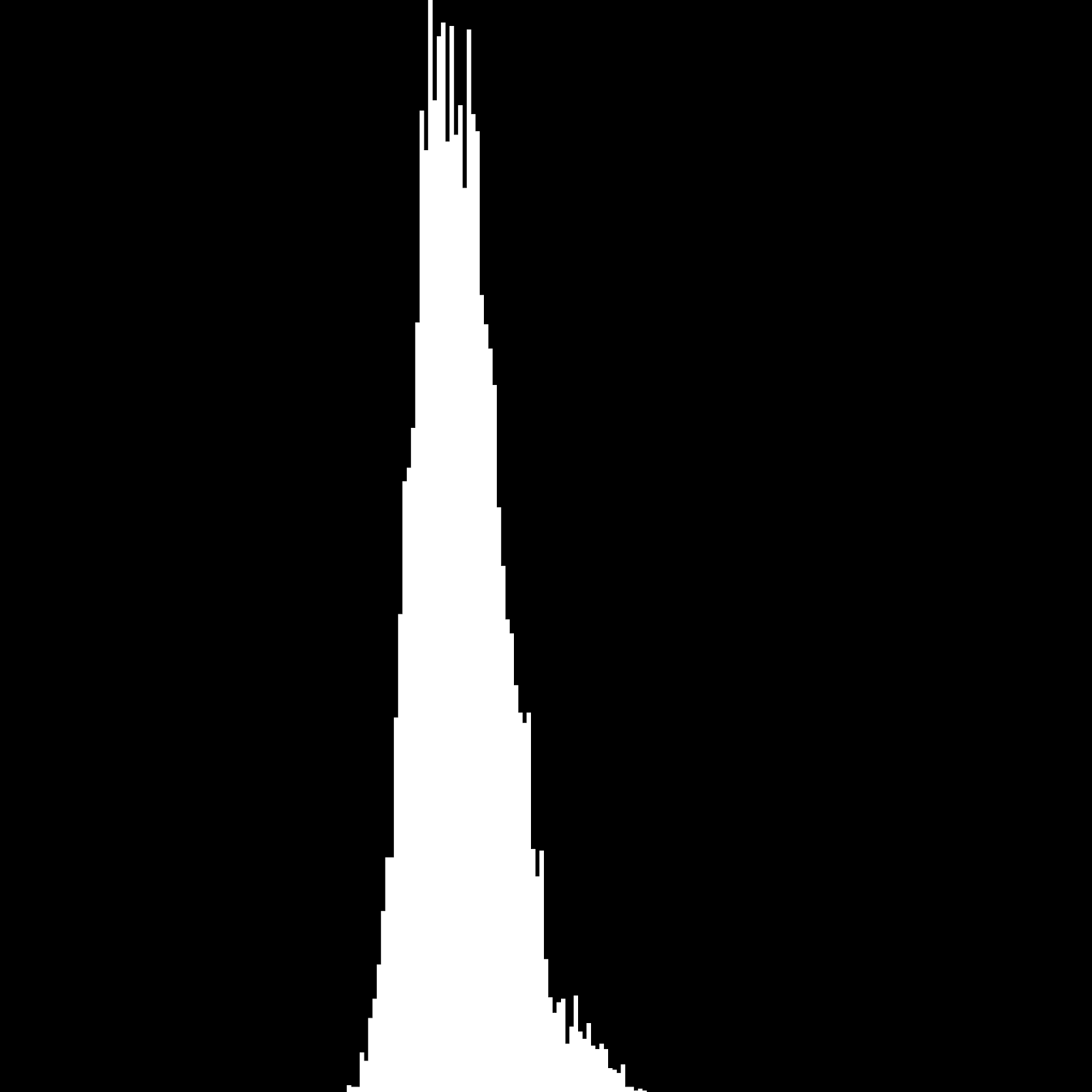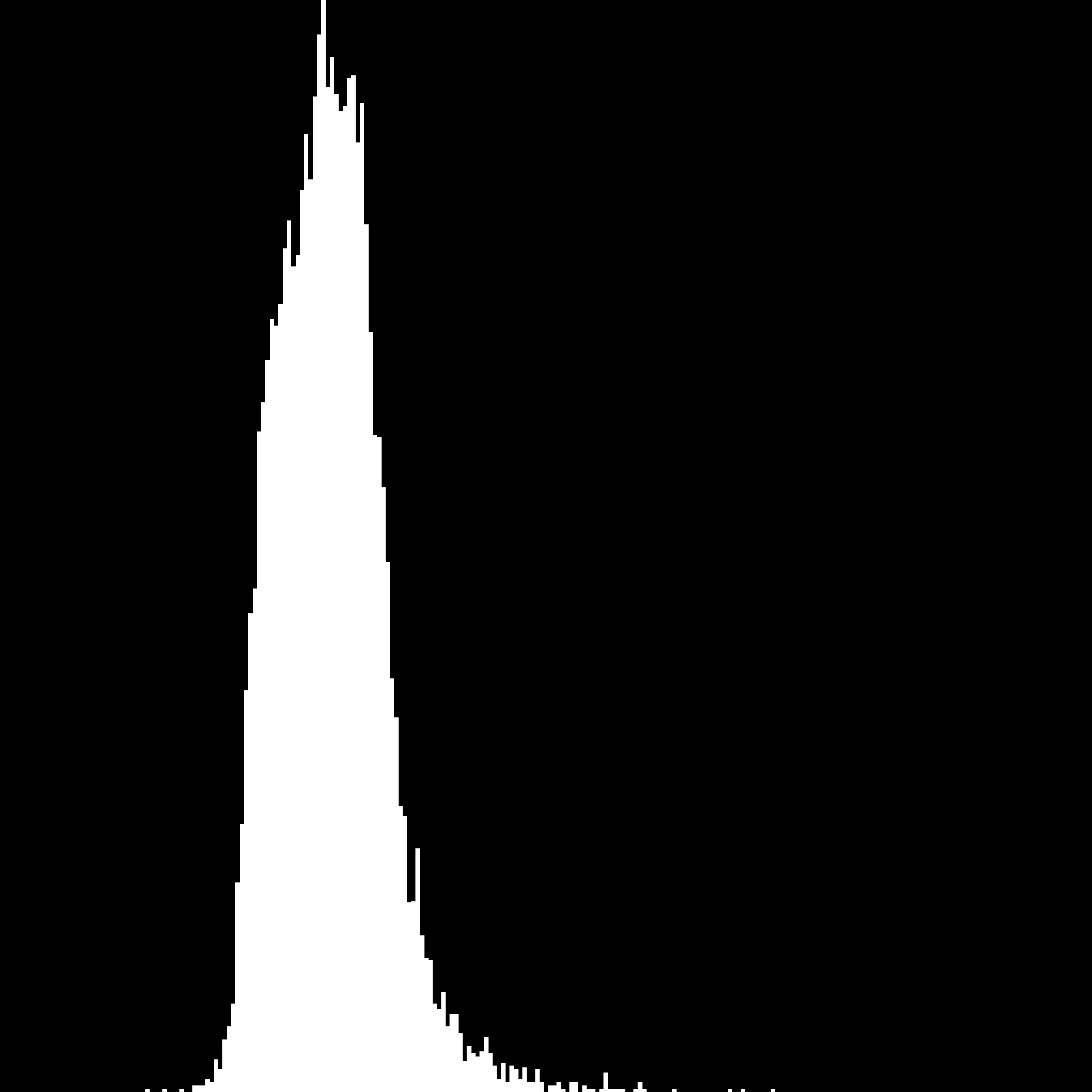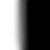2 views

I want to detect a fault in an image using logistic regression. I'm hoping to get feedback on my approach, which is as follows:

For training:

Take a small section of the image marked "bad" and "good"

Greyscale them, then break them up into a series of 5*5 pixel segments

Calculate the histogram of pixel intensities for each of these segments

Pass the histograms along with the labels to the Logistic Regression class for training

Break the whole image into 5*5 segments and predict "good"/"bad" for each segment.

Using the sigmoid function the linear regression equation is:

1/ (1 - e^(xθ))

Where x is the input values and theta (θ) is the weights. I use gradient descent to train the network. My code for this is:

void LogisticRegression::Train(float **trainingSet,float *labels, int m)

{

float tempThetaValues[m_NumberOfWeights];

for (int iteration = 0; iteration < 10000; ++iteration)

{

// Reset the temp values for theta.

memset(tempThetaValues,0,m_NumberOfWeights*sizeof(float));

float error = 0.0f;

// For each training set in the example

for (int trainingExample = 0; trainingExample < m; ++trainingExample)

{

float * x = trainingSet[trainingExample];

float y = labels[trainingExample];

// Partial derivative of the cost function.

float h = Hypothesis(x) - y;

for (int i =0; i < m_NumberOfWeights; ++i)

{

tempThetaValues[i] += h*x[i];

}

float cost = h-y; //Actual J(theta), Cost(x,y), keeps giving NaN use MSE for now

error += cost*cost;

}

// Update the weights using batch gradient desent.

for (int theta = 0; theta < m_NumberOfWeights; ++theta)

{

m_pWeights[theta] = m_pWeights[theta] - 0.1f*tempThetaValues[theta];

}

printf("Cost on iteration[%d] = %f\n",iteration,error);

}

}

Where sigmoid and the hypothesis are calculated using:

float LogisticRegression::Sigmoid(float z) const

{

return 1.0f/(1.0f+exp(-z));

}

float LogisticRegression::Hypothesis(float *x) const

{

float z = 0.0f;

for (int index = 0; index < m_NumberOfWeights; ++index)

{

z += m_pWeights[index]*x[index];

}

return Sigmoid(z);

}

And the final prediction is given by:

int LogisticRegression::Predict(float *x)

{

return Hypothesis(x) > 0.5f;

}

As we are using a histogram of intensities the input and weight arrays are 255 elements. I hope to use it on something like a picture of an apple with a bruise and use it to identify the brushed parts. The (normalized) histograms for the whole brushed and apple training sets look something like this:

For the "good" sections of the apple (y=0):For the "bad" sections of the apple (y=1):I'm not 100% convinced that using the intensities alone will produce the results I want but even so, using it on a separable data set isn't working either. To test it I passed it, labeled, completely white and a completely black image. I then run it on the small image below:Even on this image, it fails to identify any segments as being black.

Using MSE I see that the cost is converging downwards to a point where it remains, for the black and white test it starts at about cost 250 and settles on 100. The apple chunks start at about 4000 and settle in 1600.

What I can't tell is where the issues are.

Is, the approaching sound but the implementation broke? Is logistic regression the wrong algorithm to use for this task? Is gradient descent not robust enough?

by (108k points)

The problem was in your histograms which when generated weren't being memset to 0. As to the overall problem of whether or not logistic regression with grayscale images was a good solution, the answer is no. Greyscale just didn't provide enough information for good classification. Using all color channels was a bit better but I think the complexity of the problem (bruises in apples) was a bit much for simple logistic regression on its own. You can see the results on the blog here.